# How to average per month, week, or day in excel

The article will show you how to do this without going into many details. The best way to calculate these averages is by employing the use of a pivot table. The other ways will involve filtering the table and then calculating the averages one at a time, unlike pivot tables. The pivot tables will just calculate the averages per month, week, or day in excel directly without involving the many procedures. Here we go.

## How to calculate average per month or day using a pivot table

Follow these steps to calculate the average per month, week, or day in excel using the pivot table:

1. Launch excel and open the worksheet that you want to sum data in excel per month, week, or day.

2. Select all the original data tables in an open workbook.

3. Click on the Insert tab of the main menu ribbon.

4. In the Tables group, click on the From Table/Range options5. A pop-up screen PivotTable from the table or range appears. You will specify the place where the Pivot Table report will be placed. Choose New Worksheet and click OK.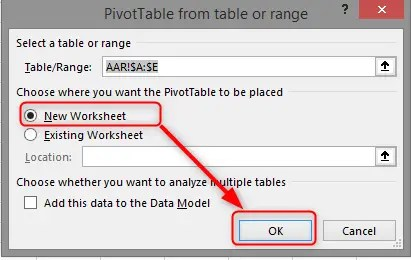6. Now Pivot Table Fields Pane is opened on a new worksheet. You will drag the Date field (a field that you want to sum by) to the Rows section alongside the Bal.Amount (the field you will sum) to the values section. Note: This can also be done by clicking the Date field and the Bal. Amount (the field you will sum) and select Add to Rows Labels and Add to Values, respectively, on the right-clicking menu.7. A table is created on the left. On the Row labels column (Date field). Select any date and right-click and select Group.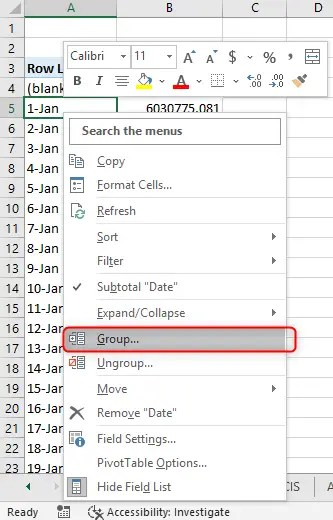8. A Grouping dialogue box will appear. Now you can proceed and choose whether to highlight a criterion you will use to Average by Box. Whether it is by day, months, or years depending on your choices. Then click OK.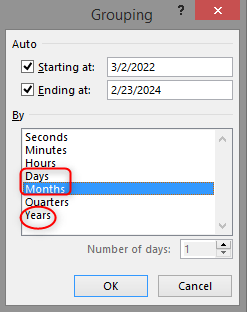9. Thereafter, in the pivot table, right-click the Sum of Bal>>Summarize Values>Average. There you each month’s averages in the Pivot Table.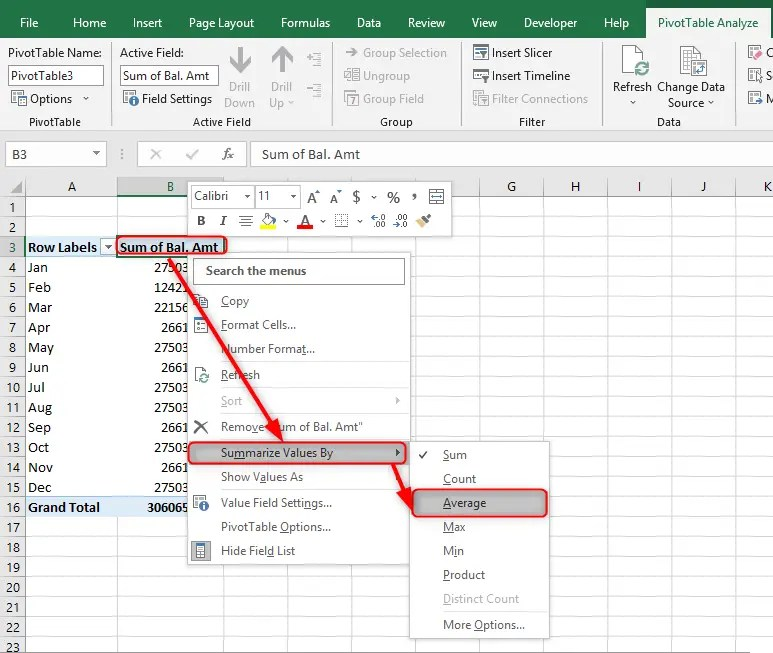10. See the example of the average per month.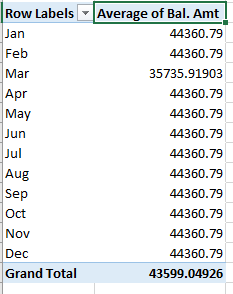## Calculating average per week using Excel Functions

Looking at the pivot table can only calculate averages for a day, month, and year. On average, on a weekly basis, you will be required to apply the Excel functions. For example, we can find the week’s average Balance Amount for the data below.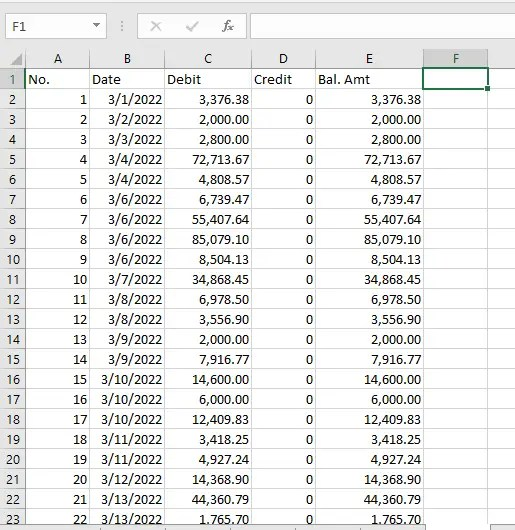1. In cell F2, we use the function =WEEKNUM(B2,2), then drag the fill handle to the bottom of the table. The function will display the week numbers in the week column.2. In cell G2, we use the function =YEAR(B2), then drag the fill handle to the bottom of the table. The function will display the year in the year column. Note: You will want to calculate the weekly average in the same year.3. In cell H2, we use the function =IF(AND(F2=F1,G2=G1),””,AVERAGEIFS(\$E\$2:\$E\$23,\$F\$2:\$F\$23,F2,\$G\$2:\$G\$23,G2)) then drag the fill handle to the bottom of the table. The function will display the average in the Average column. Note: it will calculate the weekly average in the same year while considering \$E\$2:\$E\$23 the cell to calculate the balance Amount, evaluated by week number \$F\$2:\$F\$23, and by years \$G\$2:\$G\$23. You can change the formula based on your needs F2=F1 and G2=G1 are cells with weeks and years, respectively.